## MitPY 4: Motivating Vector Products

A question from frequent commenter, Steve R:

Hi, interested to know how other teachers/tutors/academics …give their students a feel for what the scalar and vector products represent in the physical world ofandrespectively. One attempt explaining the difference between them is given here. The Australian curriculum gives a couple of geometric examples of the use of scalar product in a plane, around quadrilaterals, parallelograms and their diagonals .

Regards, Steve R

## WitCH 35: Overly Resolute

This WitCH (arguably a PoSWW) comes courtesy of Damien, an occasional commenter and an ex-student of ours from the nineteenth century. It is from the 2019 Specialist Mathematics Exam 2. We’ll confess, we completely overlooked the issue when going through the MAV solutions.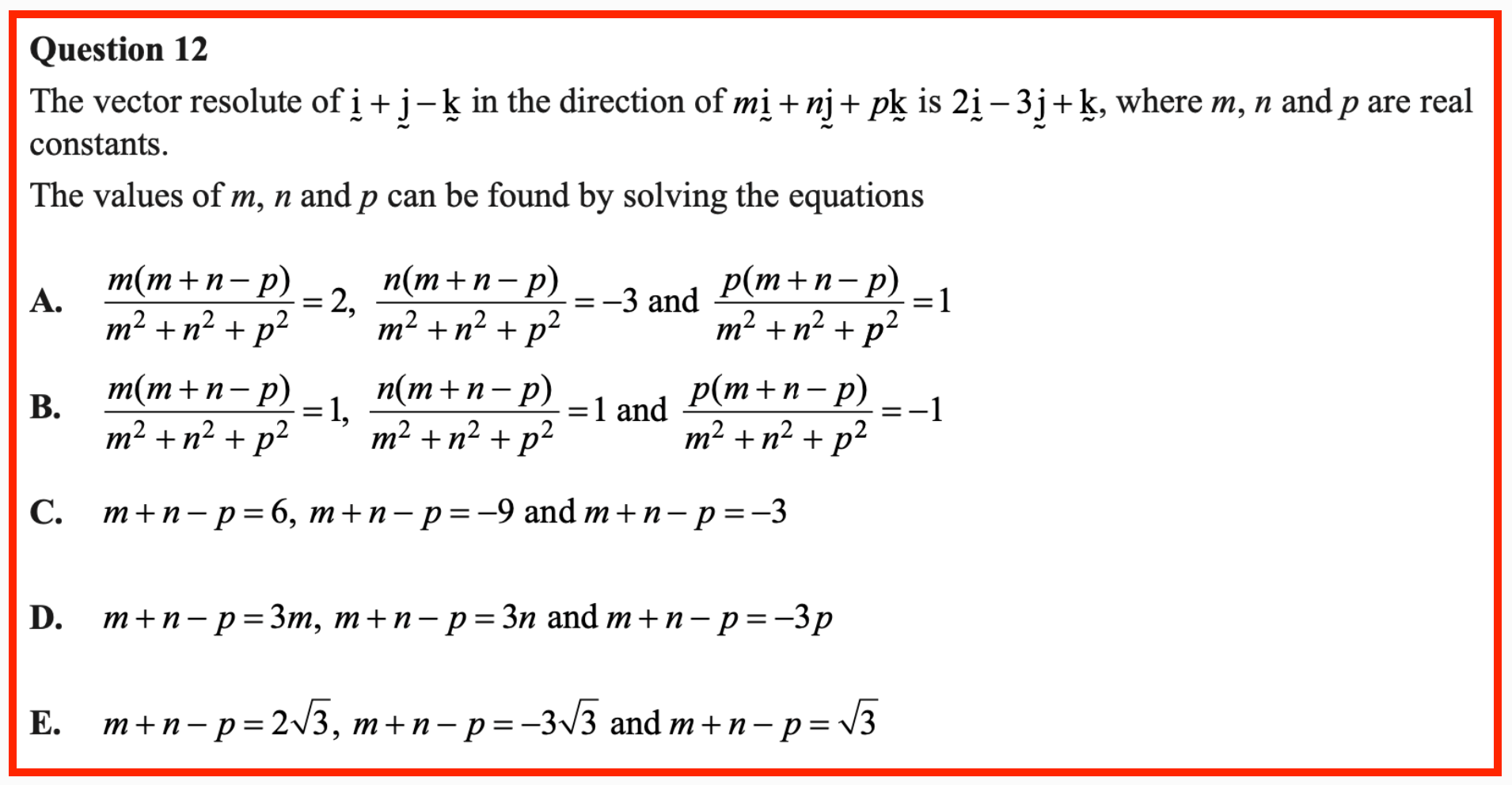Update (16/02/20)

What a mess. Thanks to Damo for pointing out the problem, and thanks to the commenters for figuring out the nonsense.

In general form, the (intended) scenario of the exam question is

The vector resolute ofin the direction ofis,

which can be pictured as follows: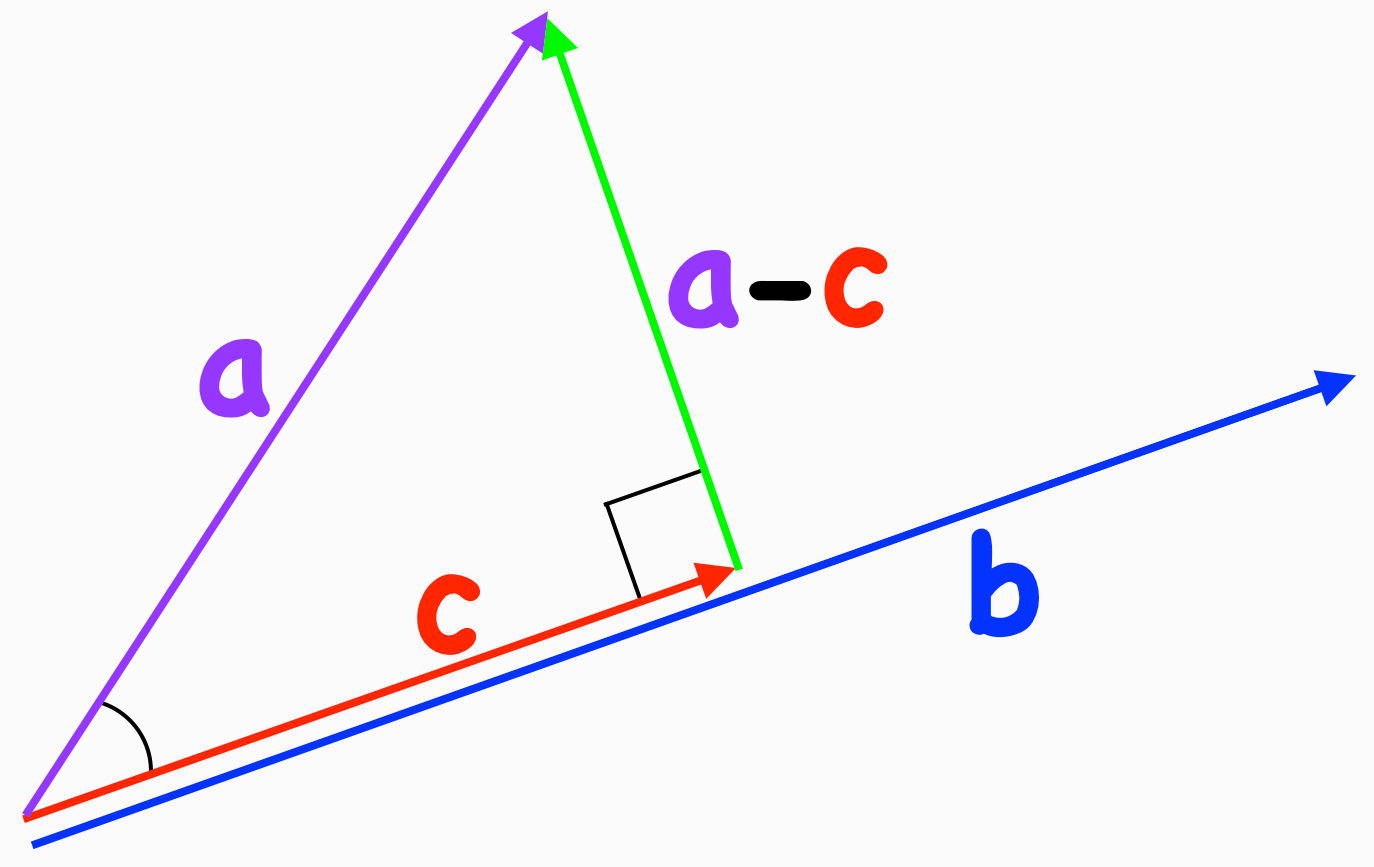For the exam question, we have,and.

Of course, givenandit is standard to find. After a bit of trig and unit vectors, we have (in most useful form)The exam question, however, is different: the question is, givenand, how to find.

The problem with that is, unless the vectorsandare appropriately related, the scenario simply cannot occur, meaningcannot exist. Most obviously, the length ofmust be no greater than the length of. This requirement is clear from the triangle pictured, and can also be proved algebraically (with the dot product formula or the Cauchy-Schwarz inequality).

This implies, of course, that the exam question is ridiculous: for the vectors in the exam we have, and that’s the end of that. In fact, the situation is more delicate; given the pictured vectors form a right-angled triangle, we require thatbe perpendicular to. Which implies, once again, that the exam question is ridiculous.

Next, suppose we lucked out and began withperpendicular to. (Of course it is very easy to check whether we’ve lucked out.) How, then, do we find? The answer is, as is made clear by the picture, “Well, duh”. The possible vectorsare simply the (non-zero) scalar multiples of, and we’re done. Which shows that the mess in the intended solution, Answer A, is ridiculous.

There is a final question, however: the exam question is clearly ridiculous, but is the question also stuffed? The equations in answer A come from the equation forabove and working backwards. And, these equations correctly return no solutions. Moreover, if the relationship betweenandhad been such that there were solutions, then the A equations would have found them. So, completely ridiculous but still ok?

Nope.

The question is framed from start to end around definite, existing objects: we have THE vector resolute, resulting in THE values of m, n and p. If the VCAA had worded the question to find possible values, on the basis of a possible direction for the resolution, then, at least technically, the question would be consistent, with A a valid answer. Still an utterly ridiculous question, but consistent. But the VCAA didn’t do that and so the question isn’t that. The question is stuffed.

Further Update (26/06/20)

As commenters have noted, the Examination Report has finally appeared. And, as predicted, answer A was deemed correct, with the Report noting

Option A gives the set of equations that can be used to obtain the values of m, n and p. Explicit solution would result in a null set as it is not possible for a result of a vector to be of greater magnitude than the vector itself.

Well, it’s something. Presumably “result of a vector” was intended to be “resolute of a vector”, and the set framing is weirdly New Mathy. But, it’s something. Seriously. As John Friend notes, it is at least a small step along the way to indicating the question is not all hunky-dory.

That step, however, is way too small. We’ll close with two comments, reiterating the points made above.

1. The question is wrong

Read the question again, and read the first sentence of the Report’s comment. The question and report justification are fundamentally stuffed by the definite articles, by the language of existence. All answers should have been marked correct.

2. The question is worse than wrong

Even if the vectorsandhad been chosen appropriately, the question is utterly devoid of mathematical sense. It suggests a long and difficult method to solve a problem that, if indeed is solvable, is trivial.

## WitCH 23: Speed Bump

Our second WitCH of the day also comes from the 2017 VCE Specialist Mathematics Exam 2. (Clearly an impressive exam, and we haven’t even gotten to the bit about using inverse trig functions to design a brooch.) It is courtesy of the mysterious SRK, who raised it in the discussion of an earlier WitCH.

Question 5 of Section B of the (CAS) exam concerns a boat and a jet ski. Though SRK was concerned with one particular aspect, the entire question is worth pondering: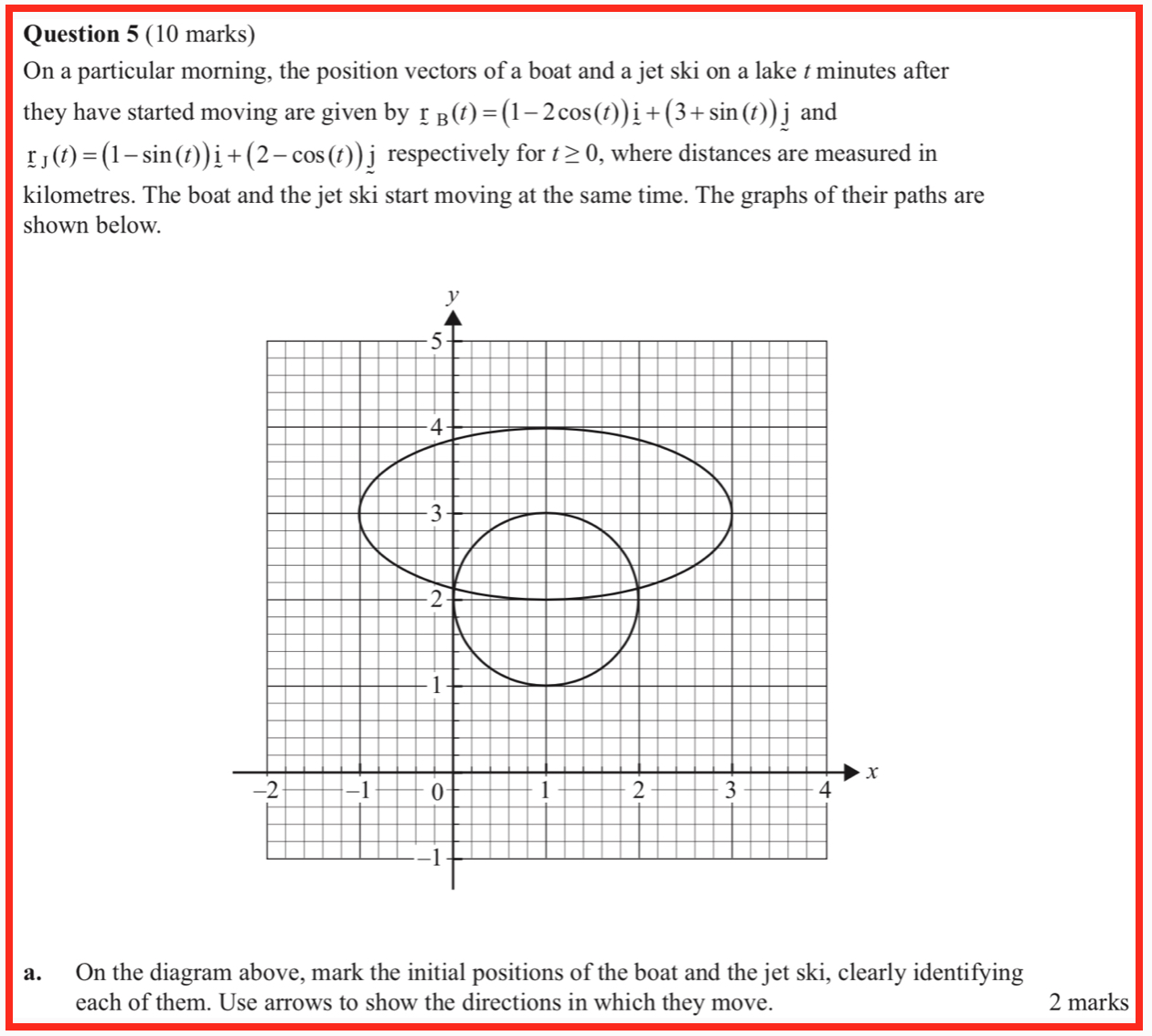The  Examiner’s Report indicates an average student score of 1.4 on part a, and comments,

Students plotted the initial positions correctly but significant numbers of students did not label the direction of motion or clearly identify the jet ski and the boat. Both requirements were explicitly stated in the question.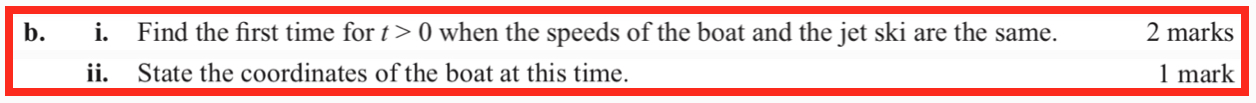For part i, the Report indicates an average score of 1.3, and comments,

Most students found correct expressions for velocity vectors. The most common error was to equate these velocity vectors rather than equating speeds.

For part ii, the Report gives the intended answer as (3,3). The Report indicates that slightly under half of students were awarded the mark, and comments,

Some answers were not given in coordinate form.For part i, the Report suggests the answer(with the displayed answer adorned by a weird, extra root sign). The report indicates that a little over half of the students were awarded the mark, and comments,

A variety of correct forms was given by students; many of these were likely produced by CAS technology, including expressions involving double angles. Students should take care when transcribing expressions from technology output as errors frequently occur, particularly regarding the number and placement of brackets. Some incorrect answers retained vectors in the expression.

For Part ii, the Report indicates the intended answer of 0.33, and that 15% of students were awarded the mark for this question. The Report comments,

Many students found this question difficult. Incorrect answers involving other locally minimum values were frequent.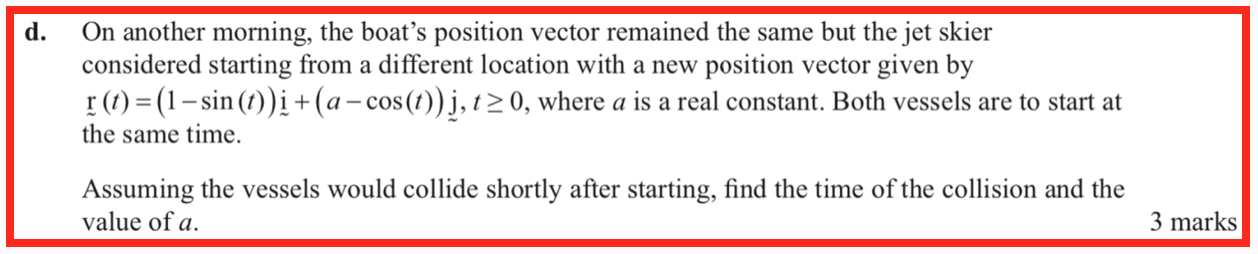The Report indicates an average score of 1.3 on part d, and comments;

Most students correctly equated the vector components and solved for t . Many went on to give decimal approximations rather than supplying the exact forms. Students are reminded of the instruction saying that an exact answer is required unless otherwise specified.

Lots there. Get hunting.

## WitCH 6: Parallel Reality

In this WitCH we will again pick on the Cambridge text Specialist Mathematics VCE Units 3 & 4 (2019): see the extract below. (We’d welcome any email or comment with suggestions of other generally WitCHful texts and/or specific WitCHes.) And, a reminder that there is still plenty left to discover in WitCH 2 , WitCH 3 and Tweel’s Mathematical Puzzle.

Have fun.

Update

Below, we go through the passage line by line, but that fails to capture the passage’s intrinsic awfulness. The passage is, as John put it pithily below, a total fatberg. The passage is worse than wrong; it is clumsy, pompous, circuitous, barely comprehensible and utterly pointless.

Why do this? Why write like this? Sure, ideas, particularly mathematical ideas, can be tricky and difficult to convey; dependence/independence isn’t particularly easy to explain. And sure, we all write less clearly than we might wish on occasion. But, if you write/proofread/edit something that the intended “readers” will obviously struggle to understand, then all you’re doing is either showing off or engaging in a meaningless ritual.

An underlying problem is that the entire VCE topic is pointless. Yes, this is the fault of the idiotic VCAA, not the text, but it has to be said, if only as a partial defence of the text. No purpose is served by including in the curriculum a subtle definition that is not then reinforced in some meaningful manner. Consequently, it’s close to impossible to cover this aspect of the curriculum in an efficient, clear and motivated manner. The text could have been one hell of a lot better, but it probably never could have been good.

OK, to the details. Grab a whisky and let’s go.

• First, a clarification. The definition of “parallel vectors” appears in a slightly earlier part of the text. We included it because it is clearly relevant to the main excerpt. We didn’t intend, however, to suggest that the discussion of dependence began with the “parallel” definition.
• For the given definition of “parallel vectors” it is redundant and distracting to specify that the scalar k not be 0.
• As discussed by Number 8, the definition of “parallel vectors” should not exclude the zero vector. The exclusion may be natural in the context of geometric proofs, but here it is a needless source of fussiness, distraction and error.  As an example of a blatant error, immediately following the above passage the text begins a proposition with “Let a and b be two linearly independent (i.e. not parallel) vectors.” A second and entirely predictable error occurs when the text later goes on to “resolve” an arbitrary vector a into components “parallel” and “perpendicular” to a second vector b.
• The definition of “linear combination” involves a clumsy and needless use of subscripts. Thankfully, though weirdly, subscripts aren’t used in the subsequent discussion. The letters used for the vector variables are changed, however, which is the kind of minor but needless, own-goal distraction that shouldn’t occur.
• No concrete example of linear combination is provided. (The more abstract the ideas, the more critical it is that they be anchored immediately with very specific illustration.)
• It is a bad choice to begin with “linear combination”. That idea is difficult enough, but it also leads to a poor and difficult definition of linear dependence, an unswallowable mouthful: “… at least one of its members [elements? vectors?] can be expressed as a linear combination of [the] other vectors [members? elements?] …” Ugh! What really kills this sentence is the “at least one”which stems from the asymmetry hiccup in the definition. (The hiccup is illustrated, for example, by the three vectors a = 3 + 2j + k, b = 9i + 6j + 3k, c = 2i + 4j + 3k. These vectors are dependent, since b = 3a + 0c is a combination of a and c. Note, however, that c cannot be written as a combination of a and b.)
• As was appropriately done for “linear combination”, the definition of linear dependence should be framed in terms of two or three vectors staring at the reader, not for “a set of vectors”.
• The language of sets is obscure and unnecessary.
• No concrete example of linear dependence is provided. There is not even the specialisation to the case of two and/or three vectors (which, again, is how they should have begun).
• If you’re going to begin with “linear combination” then don’t. But, if you are, then the definition of linear independence should precede linear dependence, since linear independence doesn’t have the asymmetry hiccup: no vector can be written as a combination of the other vectors. Then, “dependent” is defined as not independent.
• No concrete example of linear independence is provided.
• The properly symmetric “examples” are the much preferred definition(s) of dependence.
• The “For example” is weird. It is more accurate to label what follows as special cases. They are not just special cases, however, since they also incorporate non-obvious reworking of the definition of dependence.
• No proof or discussion is provided that the “example[s]”  are equivalent to the definition.
• No genuine example is provided to illustrate the “example[s]”.
• The simple identification of two vectors being parallel/non-parallel if and only if they are dependent/independent is destroyed by the exclusion of the zero vector.
• There is no indication why any set of vectors including the zero vector must be dependent.
• The expression “two-dimensional vector” is lazy and wrong: spaces have dimension, not vectors. (Ditto “three-dimensional vectors”.)
• No proof or discussion is provided that any set of three “two dimensional vectors” is dependent. (Ditto “for three-dimensional vectors”.)
• The “method” for checking the dependence of three vectors is close to unreadable. They could have begun “Let a and b be linearly independent vectors”. (Or, with the correct definition, “Let a and b be non-parallel vectors”.)
• There is no indication of or clarification of or illustration of the subtle distinction between the original “definition” of linear dependence and the subsequent “method”.

What a TARDIS of bullshit.